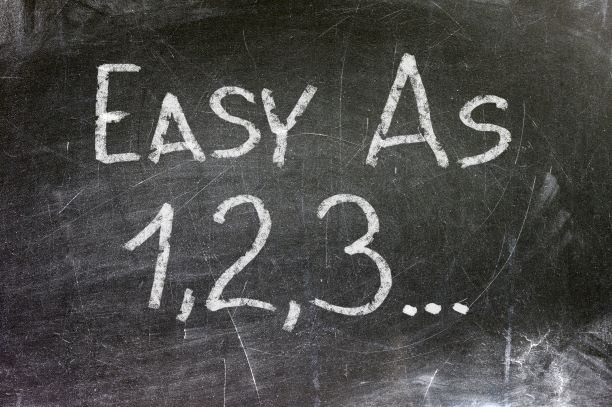# Maths whizz shares simple trick to get multiplication right every single time

If there’s one subject a lot of people really disliked at school (aside from P.E.) it was maths.
Trying to work out all those equations, fractions and percentages gave me a stonking headache and I’m sure plenty felt the same way.
But more recently, thanks to clever people on TikTok, certain maths questions aren’t as hard as they appear.
We recently came across a simple method to calculate any percentage and now we’ve found a trick to work out the answer to any number multiplied by 11.
Called the ’11’s trick’ It was shared in a video by a user named @silentmath.
In her clip, a woman (@zhcyt) is asked to quickly answer the maths problem 11 x 32.
She produces the answer 352 very fast, and according to @silentmath this is because she used the 11’s trick.
The ‘trick’ sees you separate the digits of the number you are multiplying by 11, so in this case, three and two.
You then add these numbers together, which is five (3+2=5).
This figure goes in the middle to give you the answer of 352.
Some thought they’d caught the user out, claiming the trick wouldn’t work for certain numbers – but they were proved wrong.
“What about 11 x 46, try that with this trick,” they posted. “I did it, it worked and I got 506,” was the response.
A second explained how you would solve this when the two numbers equal 10.
“Four plus six equals 10. Same happens with 11×73, seven plus three equals 10. You would just go to the next hundred, plus the last digit,” they shared.

Share